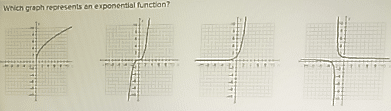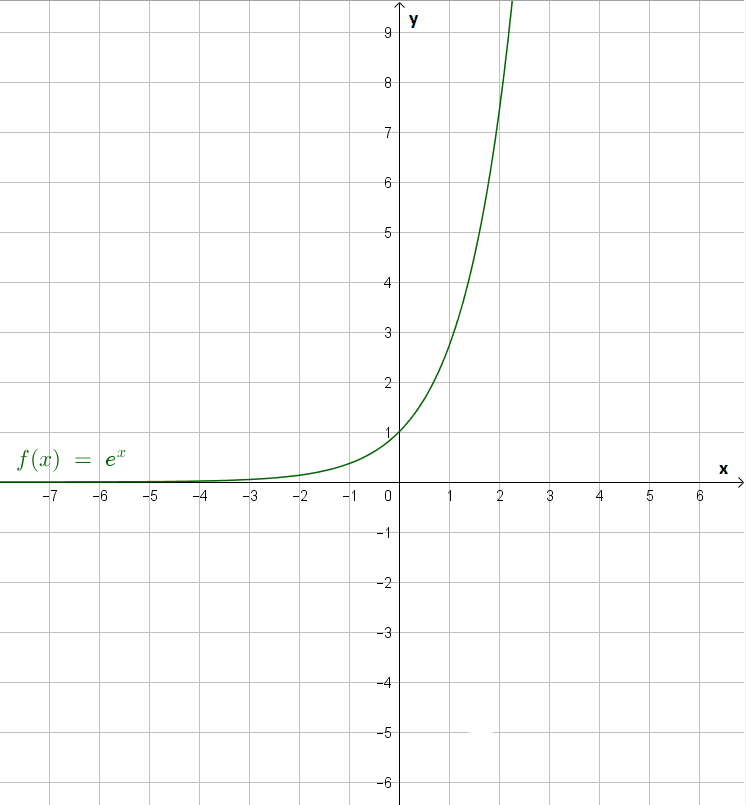# Which Graph Represents An Exponential Function

We thoroughly check each answer to a question to provide you with the most correct answers. Found a mistake? Let us know about it through the REPORT button at the bottom of the page.

Which graph represents an exponential function?## Explanation

An exponential function has the property that it cannot take on any negative values (when it has not been shifted, but when it has been shifted it still needs to be bounded horizontally). We note that the first and third graph are the only graphs that are bounded horizontally. That is, they don’t take on any negative values.

Moreover, the exponential function has the property that the function increases more and more as x increases (or the function decreases less and less as x increases when the graph has been flipped). We note that, among the first and third graph, only the third graph has this property and thus the third paragraph represents an exponential function.